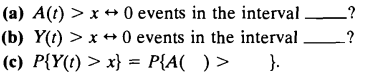### Create an Account

Home / Questions / Let A t and Y r denote the age and excess at t of a renewal-process Fill in the missing te...

# Let A t and Y r denote the age and excess at t of a renewal-process Fill in the missing terms d Compute the joint distribution of

Let A(t) and Y(r) denote the age and excess at t of a renewal-process Fill in the missing terms:(d) Compute the joint distribution of A(t) and Y(t) for a Poisson process

Aug 02 2020 View more View LessSubscribe To Get Solution